Normal view MARC view ISBD view

# Computational methods for physics / Joel Franklin, Reed College.

Material type:TextPublisher: c2013Description: xvii, 400 pages : UKP 45.00 illustrations ; 26 cm.ISBN: 9781107034303 (hardback).DDC classification: 530.15
Contents:
Machine generated contents note: 1. Programming overview; 2. Ordinary differential equations; 3. Root-finding; 4. Partial differential equations; 5. Time dependent problems; 6. Integration; 7. Fourier transform; 8. Harmonic oscillators; 9. Matrix inversion; 10. The eigenvalue problem; 11. Iterative methods; 12. Minimization; 13. Chaos; 14. Neural networks; 15. Galerkin methods; References; Index.
Summary: "There is an increasing need for undergraduate students in physics to have a core set of computational tools. Most problems in physics benefit from numerical methods, and many of them resist analytical solution altogether. This textbook presents numerical techniques for solving familiar physical problems where a complete solution is inaccessible using traditional mathematical methods. The numerical techniques for solving the problems are clearly laid out, with a focus on the logic and applicability of the method. The same problems are revisited multiple times using different numerical techniques, so readers can easily compare the methods. The book features over 250 end-of-chapter exercises. A website hosted by the author features a complete set of programs used to generate the examples and figures, which can be used as a starting point for further investigation. A link to this can be found at www.cambridge.org/9781107034303"--
Item type Current location Call number Status Date due Barcode Item holds
Book Chennai Mathematical Institute
General Stacks
530.15 FRA (Browse shelf) Available 9231
Total holds: 0
##### Browsing Chennai Mathematical Institute Shelves , Shelving location: General Stacks Close shelf browser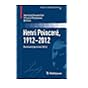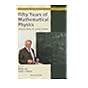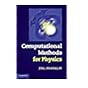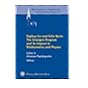No cover image available No cover image available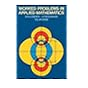530.15 DUP Henri Poincaré, 1912-2012 : Poincaré Seminar 2012 / 530.15 EBR Amplitudes, Hodge theory and ramification : from periods and motives to Feynman amplitudes : 2014 Clay Institute Summer School : periods and motives : Feynman amplitudes in the 21st century, June 30-July 25, 2014, Instituto de Ciencias Matemáticas, Madrid, Spain / 530.15 FAD Fifty years of mathematical physics : selected works of Ludvig Faddeev / 530.15 FRA Computational methods for physics / 530.15 JI Felix Klein and Sophus Lie : The Erlangen Program and its Impact in Mathematics and Physics. 530.15 JIN Classical mirror symmetry. 530.15 LEB Worked problems in applied mathematics /

Includes bibliographical references (pages 393-394) and index.

Machine generated contents note: 1. Programming overview; 2. Ordinary differential equations; 3. Root-finding; 4. Partial differential equations; 5. Time dependent problems; 6. Integration; 7. Fourier transform; 8. Harmonic oscillators; 9. Matrix inversion; 10. The eigenvalue problem; 11. Iterative methods; 12. Minimization; 13. Chaos; 14. Neural networks; 15. Galerkin methods; References; Index.

"There is an increasing need for undergraduate students in physics to have a core set of computational tools. Most problems in physics benefit from numerical methods, and many of them resist analytical solution altogether. This textbook presents numerical techniques for solving familiar physical problems where a complete solution is inaccessible using traditional mathematical methods. The numerical techniques for solving the problems are clearly laid out, with a focus on the logic and applicability of the method. The same problems are revisited multiple times using different numerical techniques, so readers can easily compare the methods. The book features over 250 end-of-chapter exercises. A website hosted by the author features a complete set of programs used to generate the examples and figures, which can be used as a starting point for further investigation. A link to this can be found at www.cambridge.org/9781107034303"--